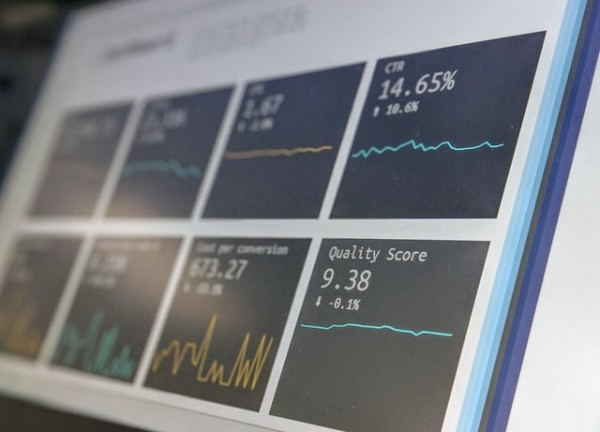# July 7th - Jad Beyhum's PhD Defense

July 07, 2020 Research

Jad Beyhum, PhD student in Mathematics at UT1-TSE, will defend his thesis on "Unobserved Heterogeneity and High-dimensional Statistics" Tuesday 7 July 2020 at 09:30 AM.

Supervisor: Eric GAUTIERMemberships are:

Abstract:

The present Thesis is composed of four articles which study different endogeneity problems in Econometrics. Endogeneity corresponds to situations where the dependence between the variable does not result from a causal relationship. These cases arise when unobserved variables called unobserved heterogeneity affect the outcome and are dependent of the regressors. The papers propose solutions using tools from the high-dimensional statistics literature. This field of research analyses estimation in the presence of a highdimensional parameter having an unknown low-dimensional underlying structure. The first paper discusses inference in a linear regression model with outliers in which the number of outliers can grow with sample size while their proportion goes to 0. The unobserved heterogeneity is here represented by an unobserved variable which value is 0 when the observation is an outlier. We propose a square-root lasso `1-norm penalized estimator, derive rates of convergence and establish asymptotic normality. Our estimator has the same asymptotic variance as the OLS estimator in the standard linear model. This enables us to build tests and confidence sets in the usual and simple manner. The second article considers a nuclear norm penalized estimator for panel data models with interactive effects, the latter constitute the unobserved heterogeneity of the model. The low-rank interactive effects can be an approximate model and the rank of the best approximation unknown and grow with sample size. An iterative procedure to compute the estimator in polynomial-time is proposed. We derive rates of convergence, study the low-rank properties of the estimator, estimation of the rank and of annihilator matrices when the number of time periods grows with the sample size. We propose and analyze a two-stage estimator and prove its asymptotic normality. None of the procedures require knowledge of the variance of the errors. The third paper considers panel data models where the dependence of the regressors and the unobservables is modelled through a factor structure. The asymptotic setting is such that the number of time periods and the sample size both go to infinity. Nonstrong factors are allowed and the number of factors can grow with the sample size. We study a class of two-step estimators of the regression coefficients. Different methods can be used in the first-step while the second-step is unique. We derive sufficient conditions on the first-step estimator and the data generating process under which the estimator is asymptotically normal. Assumptions under which using an approach based on principal components analysis in the first step yields an asymptotically normal estimator are also provided. The last article analyses the effect of a discrete treatment Z on a duration T. The treatment is not randomly assigned. The endogeneity issue is treated using a discrete instrumental variable explaining the treatment and independent of the error term of the model. Our framework is nonparametric and allows for random right-censoring. This specification generates a nonlinear inverse problem and the average treatment effect is derived from its solution. We provide local and global identification properties that rely on a nonlinear system of equations. We propose an estimation procedure to solve this system and derive rates of convergence and conditions under which the estimator is asymptotically normal. When censoring makes identification fail, we develop partial identification results. Our estimators exhibit good finite sample properties in simulations. We also apply our methodology to the Illinois Reemployment Bonus Experiment.